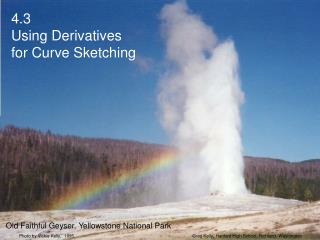DownloadDownload Presentation4.3 Using Derivatives for Curve Sketching

# 4.3 Using Derivatives for Curve Sketching

Download Presentation## 4.3 Using Derivatives for Curve Sketching

- - - - - - - - - - - - - - - - - - - - - - - - - - - E N D - - - - - - - - - - - - - - - - - - - - - - - - - - -
##### Presentation Transcript

1. Photo by Vickie Kelly, 1995 Greg Kelly, Hanford High School, Richland, Washington 4.3 Using Derivatives for Curve Sketching Old Faithful Geyser, Yellowstone National Park

2. Photo by Vickie Kelly, 2007 Greg Kelly, Hanford High School, Richland, Washington 4.3 Using Derivatives for Curve Sketching Yellowstone Falls, Yellowstone National Park

3. Photo by Vickie Kelly, 2007 Greg Kelly, Hanford High School, Richland, Washington 4.3 Using Derivatives for Curve Sketching Mammoth Hot Springs, Yellowstone National Park

4. In the past, one of the important uses of derivatives was as an aid in curve sketching. Even though we usually use a calculator or computer to draw complicated graphs, it is still important to understand the relationships between derivatives and graphs.

5. is positive is negative is zero is positive is negative is zero First derivative: Curve is rising. Curve is falling. Possible local maximum or minimum. Second derivative: Curve is concave up. Curve is concave down. Possible inflection point (where concavity changes).

6. There are roots at and . Possible extreme at . Set Example: Graph We can use a chart to organize our thoughts. First derivative test: negative positive positive

7. There are roots at and . Possible extreme at . Set maximum at minimum at Example: Graph First derivative test:

8. There is a local maximum at (0,4) because for all x in and for all x in (0,2) . There is a local minimum at (2,0) because for all x in (0,2) and for all x in . Example: Graph NOTE: On the AP Exam, it is not sufficient to simply draw the chart and write the answer. You must give a written explanation! First derivative test:

9. There are roots at and . Possible extreme at . Because the second derivative at x =0 is negative, the graph is concave down and therefore (0,4) is a local maximum. Because the second derivative at x =2 is positive, the graph is concave up and therefore (2,0) is a local minimum. Example: Graph Or you could use the second derivative test:

10. Possible inflection point at . There is an inflection point at x =1 because the second derivative changes from negative to positive. inflection point at Example: Graph We then look for inflection points by setting the second derivative equal to zero. negative positive

11. rising, concave down local max falling, inflection point local min rising, concave up Make a summary table: p